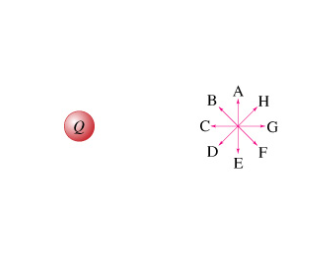# Problem: (a) If the total positive charge is Q = .62x10-6 C, what is the magnitude of the electric field caused by this charge at point P, a distance d=1.53 m from the charge?Enter your answer numerically in newtons per coulomb.(b) What is the direction of the electric field at point P?Enter the letter of the vector that represents the direction of Ep.

###### FREE Expert Solution

(a)

In this problem, we are talking about an electric field and a charge. Thus, the expression to be used is:

Electric field:

$\overline{){\mathbf{E}}{\mathbf{=}}\frac{\mathbf{k}\mathbf{q}}{{\mathbf{r}}^{\mathbf{2}}}}$

88% (306 ratings)###### Problem Details

(a) If the total positive charge is Q = .62x10-6 C, what is the magnitude of the electric field caused by this charge at point P, a distance d=1.53 m from the charge?

Enter your answer numerically in newtons per coulomb.

(b) What is the direction of the electric field at point P?

Enter the letter of the vector that represents the direction of Ep.Frequently Asked Questions

What scientific concept do you need to know in order to solve this problem?

Our tutors have indicated that to solve this problem you will need to apply the Electric Field concept. You can view video lessons to learn Electric Field. Or if you need more Electric Field practice, you can also practice Electric Field practice problems.